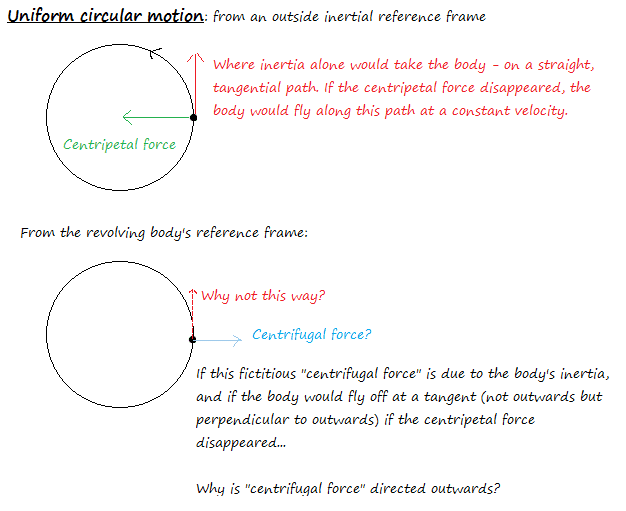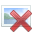# Why is 'centrifugal force' directed outwards?

My understanding is that so-called 'centrifugal force' is a fictitious force, i.e. from the reference frame of an outside (inertial) observer, there is only an inward, centripetal force on a body being accelerated in uniform circular motion, but from the reference frame of that body, objects would seem to move outwards (e.g. pendulums would swing outwards, a marble held in your hand would fly off outwards, etc.) So you have to construct a force in that reference frame, the centrifugal force, to explain that apparent outward acceleration.

However, my understanding is also that said outward motion is due to inertia (the same reason a pendulum suspended from the roof of an accelerating car flies backwards, or a person wearing roller skates on a bus, and therefore not coupled by friction to the bus floor, will roll backwards when the bus accelerates forwards. You'd have to invent fictitious forces from their reference frames to explain their apparent acceleration with respect to the car/bus too, but there is no acceleration in an inertial reference frame.) But wouldn't a body's inertia give it tangential motion, at right angles to the direction of spin rather than straight out? So why is centrifugal force (apparently) directed outwards from the centre of the circle instead of at right angles to the centre and in the direction of motion of the spinning body?

Yes, as you said inertia would keep the mody in motion along a straight line, tangetial to the circle. However, your body is forced to move on a circle which means that the direction of its velocity must change. Since you only want to change the direction of the velocity vector then you have to apply a perpendicular force directed towards the center of the circle, which is felt has a centrifugal force by the observer in the accelerated frame. Every tangent component of the force would also change the magnitude of your vector.
Is this what you where looking for?
Cheers

Ahh, of course! I didn't take into account the difference between the velocity and acceleration vector. Thank you!

rcgldr
Homework Helper
The definition of centrifugal force as used in reference to a rotating frame is independent of any actual forces applied an object that is observed from that rotating frame of reference. Centrifugal force is always (correction - added mass term m): m v^2 / r == m ω x r where ω is the angular velocity of the rotating frame, and r is the radius of the point of observation of that rotating frame. So for an object at rest to an inertial frame and also to the center of rotation of a rotating frame, the object has an outwards fictitious centrifugal force = m ω x r, and it has an inwards fictitious coriolis force = 2 m ω x r, so it appears to the observer in the rotating frame to have a net inwards centripetal force = m ω x r, even though there is no actual force at all on that object.

In the case of an object moving in circular motion relative to an inertial frame of reference, like a string exerting a centripetal force on that object, then the other part of the Newton third law pair of forces is an outwards force exerted by the object onto the string. There are exceptions to this though, like the case of two objects in a circular orbit about a common center of mass where the only forces are centripetal forces exerted by gravity on each object.

Last edited:
The definition of centrifugal force as used in reference to a rotating frame is independent of any actual forces applied an object that is observed from that rotating frame of reference. Centrifugal force is always v^2 / r == ω x r where ω is the angular velocity of the rotating frame, and r is the radius of the point of observation of that rotating frame. So for an object at rest to an inertial frame and also to the center of rotation of a rotating frame, the object has an outwards fictitious centrifugal force = ω x r, and it has an inwards fictitious coriolis force = 2 ω x r, so it appears to the observer in the rotating frame to have a net inwards centripetal force = ω x r, even though there is no actual force at all on that object.

In the case of an object moving in circular motion relative to an inertial frame of reference, like a string exerting a centripetal force on that object, then the other part of the Newton third law pair of forces is an outwards force exerted by the object onto the string. There are exceptions to this though, like the case of two objects in a circular orbit about a common center of mass where the only forces are centripetal forces exerted by gravity on each object.

Do you mean F = mv2/r = mωr? Nitpicking, I think, since for an object with constant mass it's really v (or ω) and r that matter. I know that's the value of centripetal force, anyway.

So let me see... so from an outside inertial reference frame, an object in uniform circular motion is accelerated by a centripetal force mv2/r, and that's the only force on the object (there'd be a reaction/Newton's third law force to pair with it, of course, which we could also call "centrifugal force," and it'd also be of the same magnitude, but that would be exerted by the object on something else).

I'm still confused about the fictitious centrifugal force though (the one that is only observed in the rotating body's frame of reference), and why it appears to be directed outwards if it's due to inertia. Let me explain with a diagram:I thought earlier that this is because acceleration (in the same direction as force) is the rate of change of velocity and is therefore related to the velocity vector's continuous change as the body moves round the circle. However, for an external observer, that'd just point inwards, towards the centre of the circle, and it'd just be the centripetal acceleration due to the centripetal force we already knew. And for the revolving body... there is no change of velocity, since in the body's reference frame it doesn't consider itself in acceleration - that's why we have to invent the fictitious force in the first place! So why does it appear to point outwards and not tangential? Why, if I was to bring a pendulum on a merry-go-round, would the pendulum swing outwards and not perpendicular to that?

rcgldr
Homework Helper
Do you mean F = mv2/r = mωr?
Yes, I corrected my previous post.

so from an outside inertial reference frame, an object in uniform circular motion ...
Assume the object is located as some "fixed" point on the rotating frame. The object is at "rest" within the frame, so the net force exerted on the object is zero as observed from the rotating frame. Something exerts an inwards (centripetal) force on the object, and an equal in magnitude but outwards fictitious centrifugal force is also exerted onto the object (as opposed to the real outwards force that the object exerts onto "something"), so the object remains at rest with respect to the rotating frame. (Note the Newton third law pair of forces is the inwards force that "something" exerts onto the object, and the outwards force that the object exerts onto "something". The fictitious centrifugal force exerted onto the object is not part of a Newton third law pair. If the situation was changed to an inertial frame with the force from gravity replacing the fictitious centrifugal force in the rotating frame, then the third law pair related to gravity would be the attractive gravity force from the earth exerted onto the object, and the attractive gravity force from the object exerted onto the earth).

Last edited:
A.T.